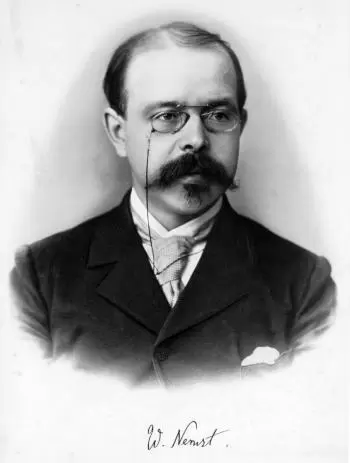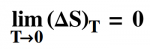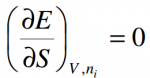# Third Law of ThermodynamicsThe third law of thermodynamics states that  absolute zero cannot be reached in a finite number of stages .

The third law of thermodynamics can also be defined as that upon reaching absolute zero, 0 degrees kelvin, any process in a physical system stops and that upon reaching absolute zero, entropy reaches a constant minimum value.

The third law of thermodynamics, sometimes called Nernst's theorem or Nernst's Postulate, relates the entropy and temperature of a physical system.

This principle states that  the entropy of a system at the temperature of absolute zero is a well-defined constant . This is because, at the absolute zero temperature, a system is in a basic state and the entropy increases are achieved by degeneration from this basic state.

Nernst's theorem states that the entropy of a perfect crystal of any element at the temperature of absolute zero is zero. However, this observation does not take into account that real crystals have been formed at temperatures above zero. Consequently, they will have defects that will not be eliminated when cooled to absolute zero. As they are not perfect crystals, the information necessary to describe the existing defects will increase the entropy of the crystal.

## Theorems and Statements of the Third Law of Thermodynamics

The most important theorems and statements related to the third law of thermodynamics are:

• Nernst's theorem.
• Nernst-Simon statement.
• Planck statement.
• Absolute Zero Inaccessibility Theorem.
• Callen's 4th Postulate.

### Nernst's Theorem

Nernst's theorem is a consequence of the third principle of thermodynamics:

A chemical reaction between crystalline pure phases that occurs at absolute zero does not produce any change in entropy. In other words, it is impossible for any process, no matter how idealized it is, to reduce the entropy of a system to its value of absolute zero in a finite number of operations.

The third law of thermodynamics allows us to find the absolute value of entropy, which cannot be done in the framework of classical thermodynamics (based on the first and second principles of thermodynamics).

Nernst's heat theorem was later used by a German physicist Max Planck to define the third law of thermodynamics in terms of entropy and absolute zero.

### Nernst-simon Statement

The change in entropy that results from any reversible isothermal transformation of a system tends to zero as the temperature approaches zero.### Planck Statement

In 1911 Max Planck formulated the third law of thermodynamics as a condition for the disappearance of the entropy of all bodies as the temperature tends to absolute zero.

Planck's formulation corresponds to the definition of entropy in statistical physics through thermodynamic probability.

According to Plank, for T → 0, the entropy of any system in equilibrium approaches a constant that is independent of the other thermodynamic variables.

### Absolute Zero Inaccessibility Theorem

The absolute zero inaccessibility theorem indicates that:

"There is no process capable of reducing the temperature of a system to absolute zero in a finite number of steps."

### callen's 4th Postulate

Callen's fourth postulate states that:

The entropy of any system is nullified in the state for which:## What Are the Consequences of the Third Principle of Thermodynamics?

The third law implies the following consequences:

1. Impossibility of reaching absolute zero temperatures
2. The behavior of thermodynamic coefficients

### Impossibility of Reaching Absolute Zero Temperatures

It follows from the third law of thermodynamics that an absolute zero temperature cannot be achieved in any final process associated with a change in entropy. It can only be addressed asymptotically.

Therefore, the third law of thermodynamics is sometimes formulated as the principle of the impossibility of reaching an absolute zero temperature.

### The Behavior of Thermodynamic Coefficients

A series of thermodynamic consequences are derived from the third law of thermodynamics: when T -> 0, it must also tend to zero:

1. the heat capacity at constant pressure and at a constant volume
2. thermal expansion coefficients and some similar values.

The validity of the third law of thermodynamics was questioned at one point, but it was later discovered that all apparent contradictions (the non-zero value of entropy in a series of substances at T = 0) are associated with metastable states of matter that cannot be considered equilibrium thermodynamically.

## References

Author:

Published: August 17, 2016
Last review: April 8, 2020